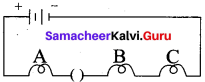You can Download Samacheer Kalvi 7th Science Book Solutions Guide Pdf, Tamilnadu State Board help you to revise the complete Syllabus and score more marks in your examinations.

## Tamilnadu Samacheer Kalvi 7th Science Solutions Term 2 Chapter 2 Electricity

### Samacheer Kalvi 7th Science Electricity Textual Evaluation

I. Choose the correct answer:

Samacheer Kalvi 7th Science Guide Term 2 Question 1.
In the circuit diagram below, 10 units of electric charge move past point x every second What is the current in the circuit ________.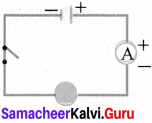(a) 10 A
(b) 1 A
(c) 10 V
(d) 1 V
(a) 10 A

Electricity Book Back Answers Question 2.
In the circuit shown, which switches (L, M or N) must be closed to light up the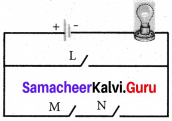(a) switch L only
(b) switch M only
(c) Switch M and N only
(d) either switch L or switches M and N
(d) either switch L or switches M and N

Samacheer Kalvi 7th Science Term 2 Question 3.
Small amounts of electrical current are measured in milliampere (mA). How many milliampere are there in 0.25 A ?
(a) 2.5 mA
(b) 25 mA
(c) 250 mA
(d) 2500 mA
(c) 250 mA

Samacheer Kalvi 7th Science 2nd Term Question 4.
which of the following circuits are the bulb connected in series?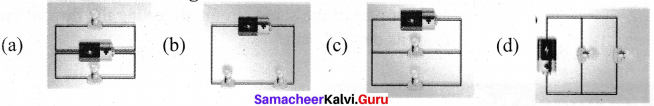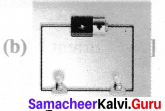II. Fill in the blanks

1. The direction of conventional current is ____________ to electron flow.
2. One unit of coulomb is charge of approximately ____________ protons or electrons.
3. ____________ is used to measure the electric current.
4. In conducting materials electrons are ____________
5. S.I. unit of Electrical conductivity of a conductor is ____________

1. Opposite
2. 6.242×10
3. Ammeter
4. Loosely
5. siemens/meters

III. True or false – If false give Hie correct answer:

Samacheer Kalvi 7th Science Book Answers Term 2 Question 1.
Electron flow is in the same direction to conventional current flow.
False.
Electron flow is in the opposite direction to conventional current flow.

7th Science Term 2 Question 2.
The fuse wire does not melts whenever there is overload in the wiring.
False.
The fuse wire melts whenever there is overload in the wiring.

7th Standard Term 2 Science Question 3.
In a parallel circuit, the electric components are divided into branches.
True.

Electricity Lesson 7th Class Question 4.
The representation of the electric current is A.
False.The representation of the electric current is I.

7th Class Electricity Lesson Question 5.
The electrical conductivity of the semiconductor is in between a conductor and an insulator.
True.

VI. Match the following :

1. Cell – used to open or close a circuit
2. Switch – safety device used in electric circuit
3. Circuit – A complete path for the flow of an electric current
4. Miniature circuit Breaker- Reset by hand, circuit becomes complete once again
5. Fuse – A device which converts chemical energy into electrical energy

1. Cell – A device which converts chemical energy into electrical energy
2. Switch – used to open or close a circuit
3. Circuit – A complete path for the flow of an electric current
4. Miniature circuit Breaker – Reset by hand, circuit becomes complete once again
5. Fuse – safety device used in electric circuit

V.Analogy:

Samacheer Kalvi Guru 7th Science Term 2 Question 1.
Water : pipe : : Electric current: ______.
wire

7th Class Science Electricity Lesson Question 2.
Copper : conductor : : Wood : ________.
insulator

7th Standard 2nd Term 2nd Lesson Question 3.
Length : meter scale : : Current : ________.
ammeter

Question 4.
milli ampere: micro ampere : : 10 – 3A: _______.
10-6A

VI. Assertion and Reason :

Question 1.
Assertion (A) : Copper1 is used to make electric wires.
Reason (R) : Copper has very low electrical resistance.
Option:
A. Both A and R are true and R is the correct explanation of A.
B. Both A and R are true but R is NOT the correct explanation of A.
C. A is true but R is false.
D. A is false but R is true.
E. Both A and R are false .
A. Both A and R are true and R is the correct explanation of A

Question 2.
Assertion (A) : Insulators do not allow the flow of current through themselves. Reason (R) : They have no free charge carriers.
A. If both A and R are true and the R is correct explanation of A.
B. If both A and R are true but R is not a correct explanation of A.
C. If A is true and R is false.
D. If both A and R are false.
A. If both A and R are true and the R is correct explanation of A

VII. Very short answer :

Question 1.
What is the speed of electric current?
Current travels at the speed of 1/100th times the speed of light 0.0002 m/s.

Question 2.
What is the S.I unit of electrical conductivity?
The SI unit of electrical conductivity is Siemens / metres (s/m).

Question 3.
Name the device used to generate electricity.
Electric cell.

Question 4.
Define fuse.
Electric fuse is a safety device which is used in household wiring and in many appliances.

Question 5.
Name some devices that run using heat effect of electric current
Electric bulb, geyser, Iron box.

Question 6.
Name few insulators.
Rubber, wood, plastic, glass.

Question 7.
What is a battery?
Batteries are a collection of one or more cells whose chemical reactions create a flow of electrons in a circuit.

VIII. Short Answer :

Question 1.
Define an electric current.
An electric current is measured by the amount of electric charge moving per unit time at any point in the circuit. The conventional symbol for current is I.

Question 2.
Differentiate parallel and serial circuits.
Parallel circuits:

1. It is connected by branches.
2. Voltage remains the same across each component of the circuit
3. Each bulb is fully powered.
4. All bulbs stay lit if one goes out.

Serial circuits:

1. It consist of single loop connection.
2. Current remains same in all parts of the circuit.
3. All the bulbs share power.
4. All bulbs go out if one goes out.

Question 3.
Define electrical conductivity.
Electrical conductivity or specific conductance is the measure of a material’s ability to conduct an electric current.

IX. Long Answer :

Question 1.
Explain flie construction and working of an Telephone.
In telephones, a changing magnetic effect causes a thin sheet of metal (diaphragm) to vibrate. The diaphragm is made up a metal that can be attracted to magnets.

1. The diaphragm is attached to spring that is fixed to the earpiece.
2. When a current flows through the wires, the soft – iron bar becomes an electromagnet.
3. The diaphragm becomes attracted to the electromagnet.
4. As the person on the other end of the line speaks, his voice cause the current in the circuit to change. This causes the diaphragm in the earpiece to vibrate, producing sound.

Question 2.
Explain the heating effect of electric current

1. When an electric current passes through a wire, the electrical energy is converted to heat.
2. In heating appliances, the heating element is made up of materials with high melting point. An example of such a material is nichrome (an alloy of nickel, iron and chromium).
3. The heating effect of electric current has many practical applications.
4. The electric bulb, geyser, iron box, immersible water heater are based on this effect.
5. These appliances have heating coils of high resistance.
6. Generation of heat due to electric current is known as the heating effect of electricity.

Question 3.
Explain the construction and working of a dry cell.

1. A dry cell is a portable form of a leclanche cell.
2. It consists of zinc vessel which acts as a negative electrode or anode.
3. The vessel contains a moist paste of saw dust saturated with a solution of ammonium chloride an chloride.
4. The ammonium chloride acts as an electrolyte.
5. The purpose of zinc pide is to maintain the moistness of the paste being highly gyroscopic.
6. The carbon rod covered with a brass cap is placed in the middle of the vessel. It acts as positive electrode or cathode.
7. It is surrounded by a closely packed mixture of charcoal and manganese dioxide (Mn02) in a muslin bag.
8. Here Mn02 acts as depolarizer. The zinc vessel is sealed at the top with pitch or shellac.
9. A small hole is provided in it to allow the gases formed by the chemical action to escape.
10. The chemical action inside the cell is the same as in avalanche cell.

X. Higher Order Question:

A student made a circuit by using an electric cell, a switch, a torch bulb (fitted in the bulb holder) and copper connecting wires. When he turned on the switch, the torch bulb did not glow at all. The student checked the circuit and found that all the wire connections were tight.

Question 1.
What could be the possible reason for the torch bulb not glowing even when the circuit appears to be complete?
The possible reasons so that even at switch ‘ON’ position the bulb is not glowing are:

1. The cell may be discharge
2. The bulb may be fused.
3. The wire may be broken from inside
4. The connection may be loose.

XI. Picture based Questions:

Question 1.
Three conductors are joined as shown in the diagram.
The current in conductor RS is 10 A. The current in conductor QR is 6 A. What will be the current in conductor PR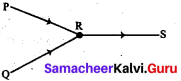(a) 4A
(b) 6 A
(c) 10 A
(d) 16 A
Solution:
PR + OR = RS
PR + 6A = 10A
PR = 10A- 6A = 4A

Question 2.
Draw the circuit diagram for the following series connection.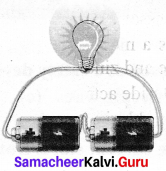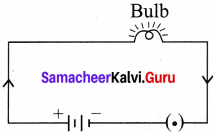Question 3.
Study the electric circuit below. Which of the following switches should be closed so that only two bulbs will light up.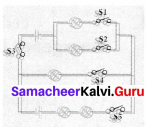(a) SI, S2 and S4 only
(b) SI, S3 and S5 only
(c) S2, S3 and S4 only
(d) S2, S3 and S5 only
(c) S2, S3 and S4 only

Question 4.
Study the three electric circuits below. Each of them has a glass rod (G), a steel rod (S), and a wooden rod (W).
In which of the electric circuits would the bulb not light up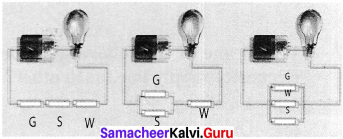(a) A only
(b) C only
(c) A and B only
(d) A, B and C
(c) A and B only

### Samacheer Kalvi 7th Science Electricity Intext Activities

Activity – 3

Question 1.
I am so exhausted. I am going to faint. What first aid will you give me to wake up?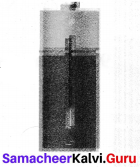I will recharge immediately

### Samacheer Kalvi 7th Science Electricity Additional Questions

I. Choose the correct answer.

Question 1.
An electric component used as a safety device is electric circuit is
(a) cell
(b) electric wires
(c) switch
(d) fuse
(d) fuse

Question 2.
In an electric circuit, the current starts from
(a) the positive terminal of the battery
(b) the negative terminal of the battery
(c) either of the terminals of the battery
(d) none
(a) the positive terminal of the battery

Question 3.
_______ was developed is 1887 by Yei Sakizo of Japan
(a) Alkaline cell
(b) Button cell
(d) Dry cell
(d) dry cell

Question 4.
________ cell is used to operate devices such as mobile phones, computers and emergency lights
(a) Primary cell Lithium cell
(b) Secondary cell
(c) Lithium Cell
(d) None
(b) Secondary cell

Question 5.
A battery is a group of _______
(a) only two cells
(c) two or more cells
(b) only single cell
(d) all of these
(c) two or more cells

Question 6.
Which of the following is an electrical conductor?
(a) cork
(b) wood
(c) plastic
(d) silver
(d) silver

Question 7.
Which one of the following phenomenon occurs when two naked wires of electricity supply line touch other?
(a) Lightning
(b) Short circuiting
(d) none
(b) Short circuiting

Question 8.
Magnetic effect of current was discovered by ____________
(a) Flemming
(b) John Dalton
(c) Newton
(d) Hans Christian Oersted
(d) Hans Christian Oersted

Question 9.
Which of the following is the symbol for a bulb?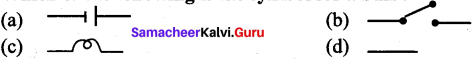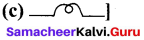Question 10.
Electricians wear rubbers gloves because it is ____________
(a) soft
(b) an insulator
(c) conductor
(d) water proof
(b) an insulator

II. Fill in the Blanks.

1. ____________ is a common example of the practical application.
2. An insulator gives a lot of ____________ to the flow of charge (electron)
3. Wires made of ____________  an electrical conductor, have very low resistance.
4. The chip which are used in SIM cards and ATM cards are made up of ____________
5. ____________  are used to remove splinters of steel or iron in hospitals dealing with eye injuries.
6. Edison used a ____________ wire coil is a vacuum glass and discovered the first electric bulb in 1879.
7. ____________ cells cannot be recharged after use. x
8. The SI unit of potential difference is____________
9. The SI unit of resistance is____________
10. An electric charge always flows from a point____________ at to a point at____________

1. Arc welding
2. resistance
3. copper
4. Semi conductors
5. Electromagnets
6. platinum
7. platinum
8. volt (V)
9. ohm
10. higher potential, lower potential

III. True or False – if false give the correct statement.

Question 1.
Insulators do not carry electric current because they do not have free electrons.
True.

Question 2.
An MCB can be used instead of a fuse in an electrical circuit.
True.

Question 3.
The flow of electric charge per unit time is called potential difference.
False. The flow of electric charge per unit time is called current.

Question 4.
The source which produce the small amount of electricity for shorter period of time is called as electro chemical cell.
True.

Question 5.
Electric cell converts electrical energy into chemical energy.
False. Electric cell converts chemical energy into electrical energy.

Question 6.
The pipe is like the wire in the electric circuit and the pump is like the battery.
True.

Question 7.
All batteries are made up of three components an anode, a cathode and some kind of electrolyte.
True.

Question 8.
An insulator gives a less resistance to the flow of charge.
False.An insulator gives a more resistance to the flow of charge.

Question 9.
Electric fuse has a body made of ceramic and two points for connecting the fuse wire.
True

Question 10.
Chemical reaction happens, when electricity passes through various conducting liquids.
True

IV. Match the following
Question 1.

1. 1 mA – (a) series
2. 1 pA – (b) ohm – metre
3. Ammeter – (c) 10-6 ampere
4. Electrical resistivity – (d) 10-3 ampere

1. (d)
2. (c)
3. (a)
4. (b)

Question 2.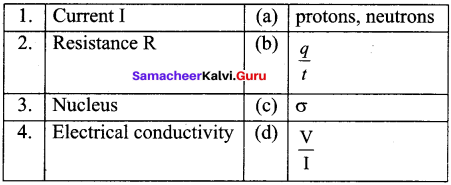1. (b)
2. (d)
3. (a)
4. (c)

Question 3.

1. Dry cells – (a) Reversible
2. Electrolyte – (b) Opens or closes the circuit
3. Switch – (c) Solution
4. Secondary cell – (d) Torches

1. (d)
2. (c)
3. (b)
4. (a)

Question 4.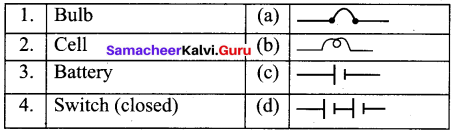1. (b)
2. (c)
3. (d)
4. (a)

V. Assertion and Reason.

Mark the correct choice as
(a) Both A and R are true but R is the correct explanation of A.
(b) Both A and R are true and R is the not the correct explanation of A.
(c) A is true but R is false.
(d) A is false but R is true.

Question 1.
Assertion (A) : Charges flow from higher potential to the lower potential.
Reason (R) : Current flows mainly due to flow of electrons.
(b) Both A and R are true and R is the not the correct explanation of A

Question 2.
Assertion (A) : Insulators do not allow flow of current through them.
Reason (R) : Insulators have no free charge carrier.
(a) Both A and R are true but R is the correct explanation of A

Question 3.
Assertion (A) : A current carrying wire should be charged.
Reason (R) : The current in a wire is due to flow of free electrons in a definite direction.
(d) A is false but R is true

Question 4.
Assertion (A) : The connecting wires are made of copper.
Reason (R) : The electrical conductivity of copper is high.
(a) Both A and R are true but R is the correct explanation of A

VI. Very short Answers:

Question 1.
What is the source of electric current in a T.V. remote?
Electric cell.

Question 2.
In which position is key placed in a circuit?
Key can be placed anywhere in the circuit.

Question 3.
What are the effects of electricity?

1. Heating effect
2. Chemical effect
3. Magnetic effect

Question 4.
Name the instrument which is used to measure potential difference.
Voltmeter.

Question 3.
Name the portable form of a avalanche cell.
Dry cell.

Question 4.
Name the substance that become ions in solutions and acquire the capacity to conduct electricity.
Electrolytes.

Question 7.
Name the component of a circuit which help us to start or stop the appliances & safely and easily.
Switch.

Question 8.
Name the component which connects electrical components to one another.
Wire.

Question 1.
What is resistance?
Resistance is the property of an electrical component which resists the flow of electric charges, when it is connected is a circuit.

Question 2.
What are the uses of electromagnets?
Electromagnets are used in cars, speakers, electric motors, electric cells, telephone receivers and television.

Question 3.
What is an electric fuse made up of?
A fuse is a small place of wire of an alloy of low melting point (63% tin and 37% lead).

Question 4.
What do you mean by magnetic effect?
When electric current passes through a wire, it behaves like a magnet. This is called
magnetic effect of electricity.

Question 5.
What are MCB’s? How do they work?

1. MCB’s (Miniature Circuit Breakers) are switches which automatically turn off when current in a circuit exceeds the safe limit.
2. We turn them on and the circuit is once again complete.

Question 6.
What is heating effect of the electric current?

1. The wire or component gets hot when an electric current passes through them,
2. This is known as heating effect of the electric current.

Question 7.
Define electric cell. What is the direction of electric current in a cell?

1. The simplest sources of an electric current is an electric cell and it has a positive terminal and a negative terminal.
2. In electric cell the current starts from positive terminal of the cell, passes through the equipment connected to it, and terminates at the negative terminal of the cell.

Question 8.
What do you mean by an open circuit?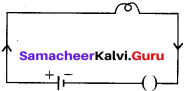An open circuit occurs when there is break in the circuit which stops the current from flowing. Breaks in the circuit can be due to many causes, such as loose bulb holder or a missing wire in the circuit.

Question 9.
Write the analogy between water current and electric current.

1. Water molecules flow towards a point of lower elevation.
2. Electric charges flow towards a point of lower voltage.

Question 10.
What are the factors affecting heating effect of electric current?

1. The amount of current I.
2. The resistance R and

Question 1.
Write the differences between primary cell and secondary cell.
Primary cell:

1. The chemical reaction inside the primary cell is irreversible
2. It cannot be recharged.
3. Examples of secondary cells are lead accumulator, Edison accumulator and Nickel – Iron accumulator.
4. Examples- simple voltalic cell, Daniel cell, and lechlanche cell and dry cell

Secondary cell:

1. The chemical reaction inside the secondary cell is reversible.
2. It can be recharged.
3. It is used to operate devices such as mobile phones, cameras, computers, and emergency lights.
4. Examples of secondary cells are lead accumulator, Edison accumulator and Nickel – Iron accumulator.

Question 2.
Explain the working of an electric fuse.

1. Electric fuse is a safety device which is used in household wing and in many appliances.
2. Electric fuse has a body made of ceramic and two points for connecting the wire.
3. The fuse wire melts whenever there is overload of the current in the wire.
4. This breaks the circuit and helps in preventing damage to costly appliances and to the wiring.
5. In electrical devices, a glass fuse is often used.
6. This is a small glass tube, in which lies the fuse wire.

Question 3.
Explain the following :

1. Semi – conductors
2. Short circuit.

1. The chip which are used in SIM Cards, Computers, and ATM cards are made up of semiconductors namely, silicon and germanium because of their electrical conductivity lies between a conductor and an insulator.

2. A short circuit is simply a low resistance connection between two conductors supplying electrical power to any circuit. Arc welding is a common example of the practical application of the heating due to a short circuit.

Question 4.
Draw the following using symbols:

1. Open circuit
2. Closed circuit
3. Series circuit
4. Parallel circuit

1. Open Circute: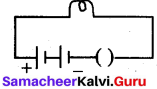2. Closed Circuit: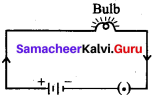3. Series Circuit: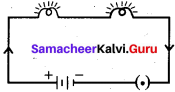4. Parallel Circuit: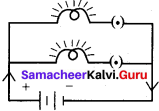IX. Problems for practice:

Question 1.
If 50 coulomb of electric charge flows through a wire in five minutes, calculate the current in the wire?
Solution:
Charge q = 50 c
time t = 5 min × 60 s = 300 s
current I = $$\frac { q }{ t }$$
= $$\frac { 50 }{ 300 }$$ = $$\frac { 5 }{ 30 }$$ = 0.166A

Question 2.
If 5A current flows through a circuit, then convert the current is terms of micro ampere?
solution :
I = 5A
IA = 106 µA
5A = 5 × 106 µA
5A = 50,00,000 µA

Question 3.
If 3A current flows through a circuit, then convert the current in terms of milliampere.
solution:
I = 3A
1A = 103 mA
3A = 3 × 1000 mA
3A = 3000 mA

X. Creative questions: HOTS

Question 1.
What is the difference between fuse wire and a heating wire?